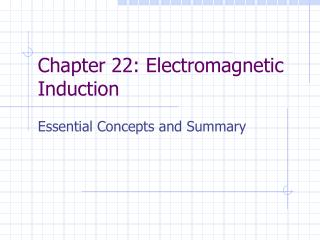DownloadDownload PresentationChapter 22: Electromagnetic Induction

# Chapter 22: Electromagnetic Induction

Télécharger la présentation## Chapter 22: Electromagnetic Induction

- - - - - - - - - - - - - - - - - - - - - - - - - - - E N D - - - - - - - - - - - - - - - - - - - - - - - - - - -
##### Presentation Transcript

1. Chapter 22: Electromagnetic Induction Essential Concepts and Summary

2. Induced EMF and Induced Current • Relative motion between magnet and coil produce an induced current • The coil behaves as if it were a source of emf, known as induced emf • Changing magnetic field induces an emf, and emf leads to an induced current • The phenomenon of producing an induced emf with the aid of a magnetic field is called electromagnetic induction

3. Motional EMF • Separated charges on the ends of the moving conductor give rise to a motional emf, existing as long as rod is moving

4. Magnetic Flux • Magnetic flux is analogous to electric flux • The quantity BA is the magnetic flux, represented by phi • Unit is tesla x meter2 (aka the Weber) • If B isn't perpendicular, however, only use the component of B that is perpendicular to compute the magnetic flux (analogous to work)

5. Faraday's Law • When there is a change in flux through a loop of wire, an emf is induced in the loop. • If it's through a coil, the induced emf is N times that induced by a single loop. • SI Unit: Volt

6. "The induced emf resulting from a changing magnetic flux has a polarity that leads to an induced current whose direction is such that the induced magnetic field opposes the original flux change" Strategy: 1. Determine if magnetic flux is increasing or decreasing 2. Find the direction of the induced field so that it opposes the flux 3. Having found the induced field, use RHR #2 to determine direction of induced current. Thus, polarity of induced current can be assigned Lenz's Law

7. Simplest form: rotating coil in uniform magnetic field This produces an alternating current. Electric motors produce back emf in proportion to their speed. Electric Generators

8. Mutual Inductance • Mutual Induction: Changing current in one circuit induces an emf in another circuit • The avg. emf in secondary coil is proportionate to change of current in primary coil. • M is the proportionality constant, known as the mutual inductance

9. Self Inductance • Self Induction: Effect in which changing current induces an emf in the same circuit • L, constant of proportionality, is the inductance, or self-inductance, of the coil

10. Energy Stored in an Inductor • Like a capacitor, an inductor can store energy • While current is rising, induced emf appears across the inductor, and thus confirming Lenz's law the polarity of the induced emf is opposite to that of the generator voltage

11. Transformers • Transformer: device for increasing or decreasing AC voltage • If a transformer steps up the voltage, the current is stepped down, conserving energy